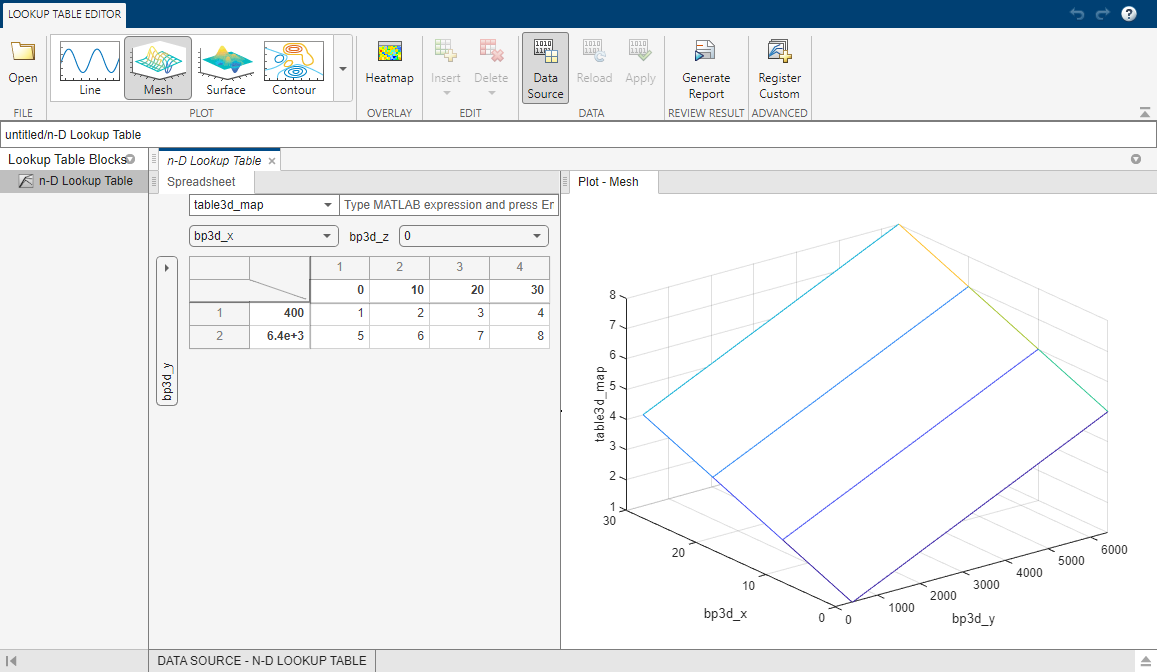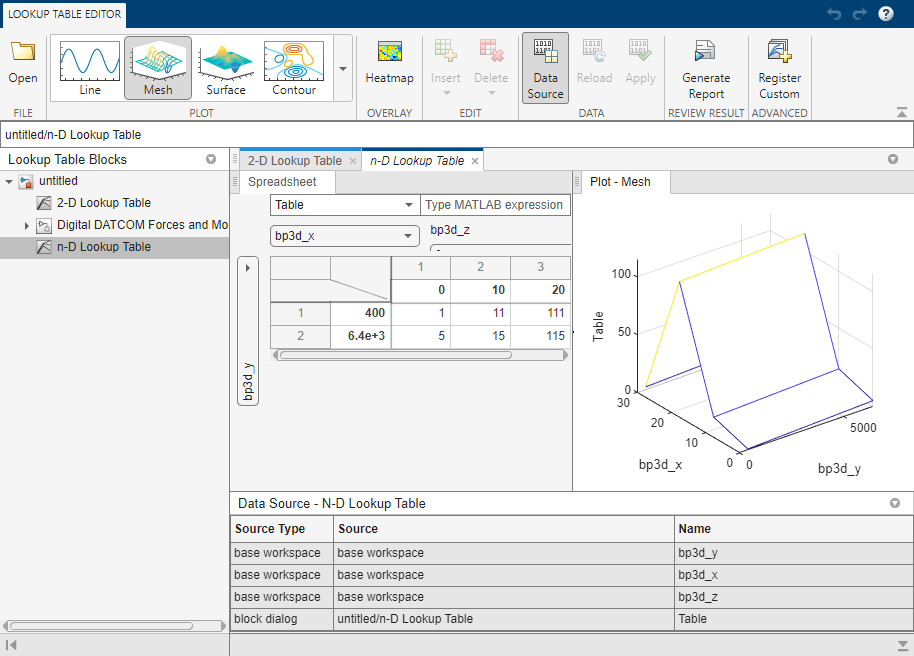## Import Lookup Table Data from MATLAB

You can import table and breakpoint data from variables in the MATLAB workspace by referencing them in the Table and Breakpoints tab of the dialog box. The following examples show how to import and export standard format and non-standard format data from the MATLAB workspace.

### Import Standard Format Lookup Table Data

Suppose you specify a 3-D lookup table in your n-D Lookup Table block.

Create workspace variables to use as breakpoint and table data for the lookup table.

```table3d_map = zeros(2,4,3); table3d_map(:,:,1) = [ 1 2 3 4; 5 6 7 8]; table3d_map(:,:,2) = [ 11 12 13 14; 15 16 17 18]; table3d_map(:,:,3) = [ 111 112 113 114; 115 116 117 118]; bp3d_z =[ 0 10 20]; bp3d_x =[ 0 10 20 30]; bp3d_y =[ 400 6400];```
Open the n-D Lookup Table block dialog box, and enter the following parameters in the Table and Breakpoints tab:

• Table data: table3d_map

• Breakpoints 1: bp3d_y

• Breakpoints 2: bp3d_x

• Breakpoints 3: bp3d_z

Click to open the Lookup Table Editor and show the data from the workspace variables.### Propagate Standard Format Lookup Table Data

When you make changes to your lookup table data, consider propagating the changes back to the MATLAB workspace variables the data was imported from using File > Update Block Data.

You can also use the Lookup Table Editor to edit the table data and breakpoint data set of `Simulink.LookupTable` and the breakpoint data set of `Simulink.Breakpoint` objects and propagate the changes back to the object.

Suppose you make a change to the lookup table variables imported from the MATLAB workspace variables in Import Standard Format Lookup Table Data. For example, change the value of the data in (1,1,1) from `1` to `33`. To propagate this change back to `table3d_map` in the workspace, in the Lookup Table Editor toolstrip, click Apply.

### Import Nonstandard Format Lookup Table Data

Suppose you specify a 3-D lookup table in your n-D Lookup Table block. Create workspace variables to use as breakpoint and table data for the lookup table. The variable for table data, `table3d_map_custom`, is a two-dimensional matrix.

```table3d_map_custom = zeros(6,4); table3d_map_custom = [ 1 2 3 4; 5 6 7 8; 11 12 13 14; 15 16 17 18; 111 112 113 114; 115 116 117 118]; bp3d_z =[ 0 10 20]; bp3d_x =[ 0 10 20 30]; bp3d_y =[ 400 6400];```
Open the n-D Lookup Table block dialog box, and enter the following parameters in the Table and Breakpoints tab. Transform `table3d_map_custom` into a three-dimensional matrix for the table data input using the `reshape` command.

• Table data: `reshape(table3d_map_custom,[2,4,3])`

• Breakpoints 1: `bp3d_y`

• Breakpoints 2: `bp3d_x`

• Breakpoints 3: `bp3d_z`

Click to open the Lookup Table Editor and show the data from the workspace variables.Change 1 to 33 in the Lookup Table Editor. The Lookup Table Editor records your changes by maintaining a copy of the table. To restore the variable values from the MATLAB® workspace, in the Lookup Table Editor toolstrip, click Reload. To update the MATLAB workspace variables with the edited data, in the Lookup Table Editor toolstrip, click Apply. You cannot propagate the change to `table3d_map_custom`, the workspace variable that contains the nonstandard table data for the n-D Lookup Table block. To propagate the change, you must register a customization function that resides on the MATLAB search path. For details, see Propagate Nonstandard Format Lookup Table Data.

### Propagate Nonstandard Format Lookup Table Data

This example shows how to propagate changes from the Lookup Table Editor to workspace variables of nonstandard format. Suppose your Simulink® model from Import Nonstandard Format Lookup Table Data has a three-dimensional lookup table that gets its table data from the two-dimensional workspace variable `table3d_map_custom`. Update the lookup table in the Lookup Table Editor and propagate these changes back to `table3d_map_custom` using a customization function.

1. Create a file named `sl_customization.m` with these contents.

```function sl_customization(cm) cm.LookupTableEditorCustomizer.getTableConvertToCustomInfoFcnHandle{end+1} = ... @myGetTableConvertInfoFcn; end```

In this function:

• The argument `cm` is the handle to a customization manager object.

• The handle `@myGetTableConvertInfoFcn` is added to the list of function handles in the cell array for `cm.LookupTableEditorCustomizer.getTableConvertToCustomInfoFcnHandle`. You can use any alphanumeric name for the function whose handle you add to the cell array.

2. In the same file, define the `myGetTableConvertInfoFcn` function.

```function blkInfo = myGetTableConvertInfoFcn(blk,tableStr) blkInfo.allowTableConvertLocal = true; blkInfo.tableWorkSpaceVarName = 'table3d_map_custom'; blkInfo.tableConvertFcnHandle = @myConvertTableFcn; end```

The `myGetTableConvertInfoFcn` function returns the `blkInfo` object containing three fields.

• `allowTableConvertLocal` — Allows table data conversion for a block.

• `tableWorkSpaceVarName` — Specifies the name of the workspace variable that has a nonstandard table format.

• `tableConvertFcnHandle` — Specifies the handle for the conversion function.

When `allowTableConvertLocal` is set to `true`, the table data for that block is converted to the nonstandard format of the workspace variable whose name matches `tableWorkSpaceVarName`. The conversion function corresponds to the handle that `tableConvertFcnHandle` specifies. You can use any alphanumeric name for the conversion function.

3. In the same file, define the `myConvertTableFcn` function. This function converts a three-dimensional lookup table of size Rows * Columns * Height to a two-dimensional variable of size (Rows*Height) * Columns.

```% Converts 3-dimensional lookup table from Simulink format to % nonstandard format used in workspace variable function cMap = myConvertTableFcn(data) % Determine the row and column number of the 3D table data mapDim = size(data); numCol = mapDim(2); numRow = mapDim(1)*mapDim(3); cMap = zeros(numRow, numCol); % Transform data back to a 2-dimensional matrix cMap = reshape(data,[numRow,numCol]); end```
4. Put `sl_customization.m` on the MATLAB search path. You can have multiple files named `sl_customization.m` on the search path. For more details, see Behavior with Multiple Customization Functions.

5. Refresh Simulink customizations at the MATLAB command prompt.

`sl_refresh_customizations`
6. Open the Lookup Table Editor for your lookup table block and select File > Update Block Data. Click to overwrite the workspace variable `table3d_map_custom`.

7. Check the value of `table3d_map_custom` in the base workspace.

```table3d_map_custom = 33 2 3 4 5 6 7 8 11 12 13 14 15 16 17 18 111 112 113 114 115 116 117 118```

The change in the Lookup Table Editor has propagated to the workspace variable.

Note

If you do not overwrite the workspace variable `table3d_map_custom`, you are prompted to replace it with numeric data. Click to replace the expression in the Table data field with numeric data. Click if you do not want your Lookup Table Editor changes for the table data to appear in the block dialog box.

#### Behavior with Multiple Customization Functions

At the start of a MATLAB session, Simulink loads each `sl_customization.m ` customization file on the path and executes the `sl_customization` function. Executing each function establishes the customizations for that session.

When you select File > Update Block Data in the Lookup Table Editor, the editor checks the list of function handles in the cell array for `cm.LookupTableEditorCustomizer.getTableConvertToCustomInfoFcnHandle`. If the cell array contains one or more function handles, the `allowTableConvertLocal` property determines whether changes in the Lookup Table Editor can be propagated.

• If the value is set to `true`, then the table data is converted to the nonstandard format in the workspace variable.

• If the value is set to `false`, then table data is not converted to the nonstandard format in the workspace variable.

• If the value is set to `true` and another customization function specifies it to be `false`, the Lookup Table Editor reports an error.